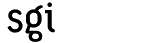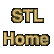# powerCategory: algorithms Component type: function

### Prototype

Power is an overloaded name; there are actually two power functions.
```template <class T, class Integer>
inline T power(T x, Integer n);

template <class T, class Integer, class MonoidOperation>
T power(T x, Integer n, MonoidOperation op);
```

### Description

Power is generalized exponentiation: it raises the value x to the power n, where n is a non-negative integer.

The first version of power returns x raised to the nth power; that is, it returns x * x ... * x, where x is repeated n times.  If n == 0, then it returns identity_element(multiplies<T>()).

The second version of power is just like the first except that it uses an arbitrary Monoid Operation instead of multiplies<T>, returning identity_element(op) when n == 0.

Important note: power does not assume that multiplication is commutative, but it does rely crucially on the fact that multiplication is associative. If you have defined * or MonoidOperation to be a non-associative operation, then power will give you the wrong answer. 

### Definition

Defined in the standard header numeric, and in the nonstandard backward-compatibility header algo.h. This function is an SGI extension; it is not part of the C++ standard.

### Requirements on types

For the first version:
For the second version:
• MonoidOperation is a model of Monoid Operation.
• T is MonoidOperation's argument type.
• n is an integral type.

• n >= 0.

### Complexity

The number of multiplications (or, in the case of the second version, the number of applications of MonoidOperation) is lg(n) + nu(n) where lg is the base 2 logarithm and nu(n) is the number of 1s in the binary representation of n. 

### Example

```int main() {
cout << "2 ** 30 = " << power(2, 30) << endl;
}
```

### Notes

 This is a conceptual description of what power's return value is; it is not how power is actually implemented. If power were implemented that way then it would require n-1 multiplications, which would be grossly inefficient. Power is implemented using the "Russian peasant algorithm", which requires only O(log n) multiplications. See section 4.6.3 of Knuth (D. E. Knuth, The Art of Computer Programming. Volume 2: Seminumerical Algorithms, Addison-Wesley, 1981) for a discussion.

 See the Monoid Operation requirements for a discussion of associativity.

 This is in fact not the minimum possible number of multiplications: it is possible to compute the fifteenth power of x using only five multiplications, but power(x, 15) uses six.